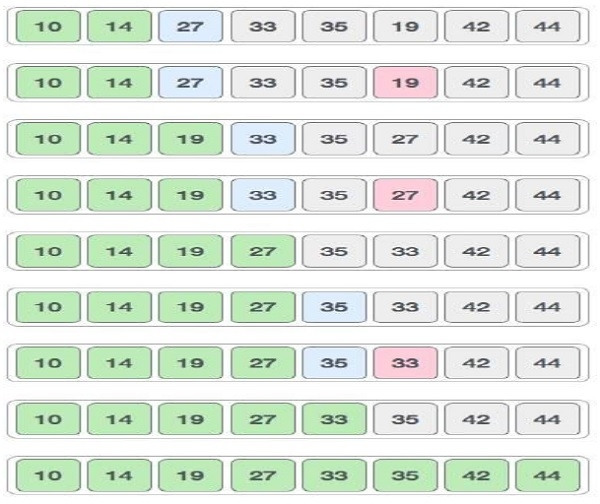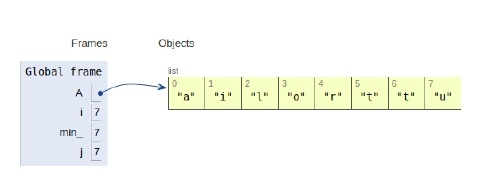# Python Program for Selection Sort

In this article, we will learn about the Selection sort and its implementation in Python 3.x. Or earlier.

In the selection sort algorithm, an array is sorted by recursively finding the minimum element from the unsorted part and inserting it at the beginning. Two subarrays are formed during the execution of Selection sort on a given array.

• The subarray, which is already sorted
• The subarray, which is unsorted.

During every iteration of selection sort, the minimum element from the unsorted subarray is popped and inserted into the sorted subarray.

Let’s see the visual representation of the algorithm −Now let’s see the implementation of the algorithm −

## Example

Live Demo

A = ['t','u','t','o','r','i','a','l']
for i in range(len(A)):
min_= i
for j in range(i+1, len(A)):
if A[min_] > A[j]:
min_ = j
#swap
A[i], A[min_] = A[min_], A[i]
# main
for i in range(len(A)):
print(A[i])

## Output

a
i
l
o
r
t
t
u

Here we received output from the algorithm in ascending order. Min_ is the current value which is getting compared with all other values. The analysis parameters of the algorithm are listed below −

Time Complexity − O(n^2)

Auxiliary Space − O(1)

Here all the variables are declared in the global frame as shown in the image below